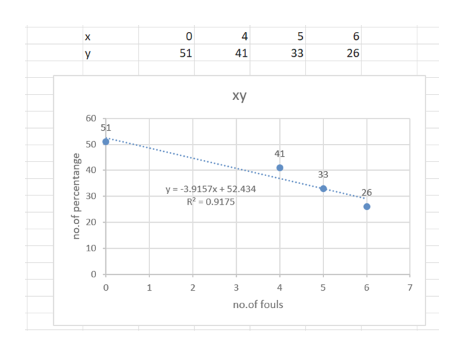Question
3 views

It is thought that basketball teams that make too many fouls in a game tend to lose the game even if they otherwise play well. Let x be the number of fouls more than (i.e., over and above) the opposing team. Let y be the percentage of times the team with the larger number of fouls wins the game.

 x 0 4 5 6 y 51 41 33 26

Complete parts (a) through (e), given Σx = 15, Σy = 151, Σx2 = 77, Σy2 = 6047, Σxy = 485, and

r ≈ −0.958.
(a) Draw a scatter diagram displaying the data.

Flash Player version 10 or higher is required for this question.
You can get Flash Player free from Adobe's website.

(b) Verify the given sums Σx, Σy, Σx2, Σy2, Σxy, and the value of the sample correlation coefficient r. (Round your value for r to three decimal places.)
 Σx = Σy = Σx2 = Σy2 = Σxy = r =

(c) Find x, and y. Then find the equation of the least-squares line  = a + bx. (Round your answers for x and y to two decimal places. Round your answers for a and b to three decimal places.)
 x = y = = +  x

(d) Graph the least-squares line. Be sure to plot the point (x, y) as a point on the line.
(e) Find the value of the coefficient of determination r2. What percentage of the variation in y can be explained by the corresponding variation in x and the least-squares line? What percentage is unexplained? (Round your answer for r2 to three decimal places. Round your answers for the percentages to one decimal place.)
 r2 = explained % unexplained %

(f) If a team had x = 3 fouls over and above the opposing team, what does the least-squares equation forecast for y? (Round your answer to two decimal places.)
%
check_circle

1. Scatter diagram....help_outlineImage Transcriptionclose4 6 х 33 26 51 41 ху 60 51 50 41 40 33 y3.9157x+52.434 R2-0.9175 26 30 20 10 0 1 2 5 6 7 no.of fouls Ln m O Ln no.of percentange fullscreen

### Want to see the full answer?

See Solution

#### Want to see this answer and more?

Solutions are written by subject experts who are available 24/7. Questions are typically answered within 1 hour.*

See Solution
*Response times may vary by subject and question.
Tagged in

### Statistics